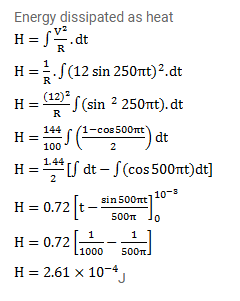# A resistor of resistance 100Ω is connected to an AC

Question:

A resistor of resistance $100 \Omega$ is connected to an $\mathrm{AC}$ source $^{\varepsilon}=(12 \mathrm{~V}) \sin \left(250 \pi \mathrm{s}^{-1}\right) \mathrm{t}$. Find the energy dissipated as heat during $\mathrm{t}=0$ to $\mathrm{t}=0.1 \mathrm{~ms}$.

Solution: